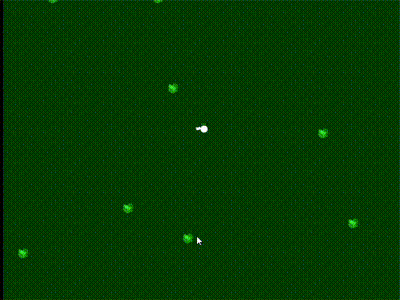-=+=- -=+=- -=+=- -=+=- -=+=- -=+=- -=+=- -=+=- -=+=- -=+=- -=+=- -=+=- -=+=- -=+=- -=+=- -=+=- -=+=- -=+=- -=+=- -=+=- -=+=- -=+=- -=+=- -=+=- -=+=- -=+=- -=+=- -=+=- -=+=- -=+=- (c) WidthPadding Industries 1987 0|58|0 -=+=- -=+=- -=+=- -=+=- -=+=- -=+=- -=+=- -=+=- -=+=- -=+=- -=+=- -=+=- -=+=- -=+=- -=+=- -=+=- -=+=- -=+=- -=+=- -=+=- -=+=- -=+=- -=+=- -=+=- -=+=- -=+=- -=+=- -=+=- -=+=- -=+=-
SoCoder -> Showcase Home -> Remakes

PakzCreated : 16 March 2018
Edited : 21 January 2019
System : Browser(emscripten)
Language : Monkey2

### Fire Power

Fire Power

mx2 - Source code
ScreenshotsI started this a while ago. The original game was a Amiga and Dos game.

It was one of those games I played with my brother when we had 2 Amiga's connected together.

The original game is located on a top down 2d map. There are two bases. Each player controls a tank. There are turrets in each base that attacks the players tanks. You can destroy the turrets and walls. There are buildings that can also be destroyed. Little people run out and they can be shot or run over.
The goal is to capture the flag and return it to your base. You can also free pows and transport them back to your base. There is also a helicopter for each side that attacks the tanks. They are not controllable.

For the remake I am planning a different control method for the tanks. In the original game it was kind of weird. Multi player might not be done or maybe split screen. Maybe if there are some people who start playing it and contact me for testing then I might experiment with real multiplayer. The game will be open sourced so anyone could add this themselfs also.

## Latest Update

Currently working on remaking the graphics. I am using no external files and doing everything by code. I have not recreated a lot yet but I am at a thousand lines already.

There is no playable anything yet. Just the code for graphics. I need to recreate a certain amount of graphics and then I will start on some kind of editor and map generator. Also I have been thinking on how to program the tanks.

I still need to buy the original amiga kickstart roms since firepower does not seem to work with the free amiga rom. I am using video of the game and pictures right now.
Edit :
I just bought the amiga forever package. The game worked straight away and have played it for a while already. The controls are not as bad as I thought. I might just reprogram it.

Last ingame video :